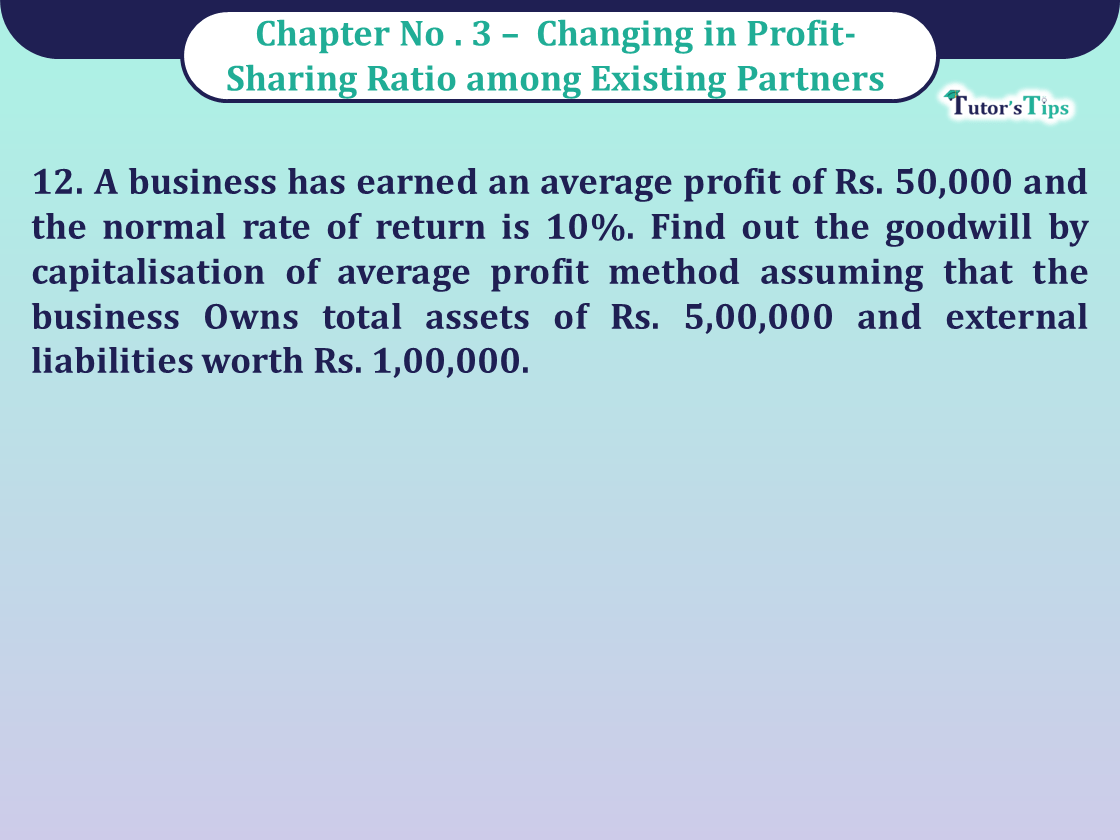# Question 12 Chapter 3 of Class 12 Part – 1 VK PublicationQuestion 12 Chapter 3 of Class 12 Part - 1 VK Publication

Question 12 Chapter 3 of Class 12 Part – 1

12. A business has earned an average profit of Rs. 50,000 and the normal rate of return is 10%. Find out the goodwill by capitalisation of average profit method assuming that the business Owns total assets of Rs. 5,00,000 and external liabilities worth Rs. 1,00,000.

## The solution of Question 12 Chapter 3 of Class 12 Part – 1: –

12. Capital Employed or Net Asset of the firm= Total Assets – External Liability

= 5,00,000-1,00,000 = Rs. 4,00,000

 Capitalised Value of the firm= Average profit X 100 Normal Rate of Return
 = 50,000 X 100 10

= Rs.5,00,000

Goodwill= Capitalised value of the Firm – Capital Employed
= 5,00,000-4,00,000 = Rs. 1,00,000

Comment if you have any questions.

Also, Check out the solved question of previous Chapters: –